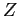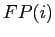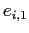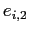Next: Unsupervised classification by OPF Up: Supervised classification by OPF Previous: Supervised classification by OPF

### Accuracy measure

The accuracy of a classifier can be measured in any set: training, evaluation, and test. Letbe any one of these sets andbe the number of samples in. The accuracyis measured by taking into account that the classes may have different sizes in. If there are two classes, for example, with very different sizes and a classifier always assigns the label of the largest class, its accuracy will fall drastically due to the high error rate on the smallest class.

Let,, be the number of samples infrom each class. We defineand(1)

whereandare the false positives and false negatives, respectively. That is,is the number of samples from other classes that were classified as being from the classin, andis the number of samples from the classthat were incorrectly classified as being from other classes in. The errorsandare used to define(2)

whereis the partial sum error of class. Finally, the accuracyof the classification is written as(3)Next: Unsupervised classification by OPF Up: Supervised classification by OPF Previous: Supervised classification by OPF
Joao Paulo Papa 2009-09-30